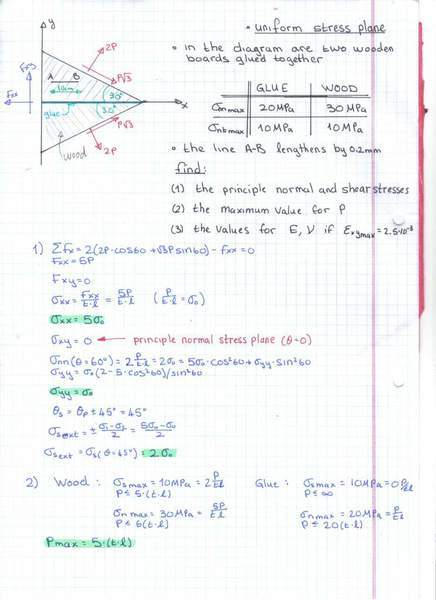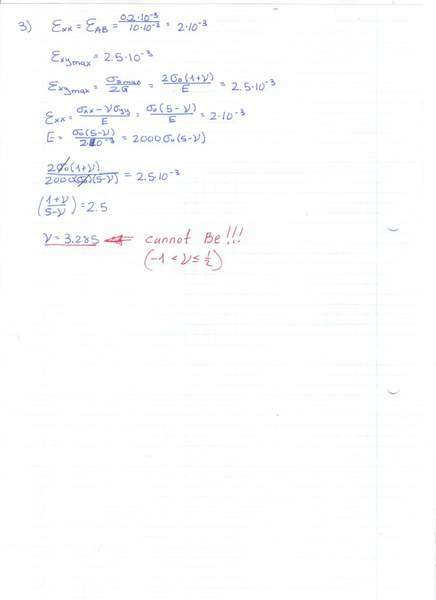# Given 2 wooden triangular boards, glued together in their centres,

given 2 wooden triangular boards, glued together in their centres, find
1)the principle stresses
2)the maximum value for P
3) the values for V and E

the given values are in the scanned images
all my calculations are in the scanned images

the question and calculations for 1,21) i first looked for the total normal and shear force by "adding" Fxx and Fxy on the left hand side
after finding the forces i found the stresses,
i calculated the shear stress xy to be 0, therefore the x'y plane is the principle normal stress plane and 45 degrees to it will be the principle shear plane.
using the tensor transformation formula i found the normal stress yy

the length of a side of the triangle was not given so i called it L; the thickness of the board was also not given, i called it t, to simplify calculations i called P/(L*t)=simga-o
could not manage to cancel out the parameters i added, L and t

2) to find the maximum value of P, i compared the shear and normal stresses in the appropriate directions to the maximum values for the wood and the glue, i chose the minimum value for P as my maximum allowed P

3) for the 3rd questionm i could not get a logical answer!!
also, is WOOD elastic?? can i even use hookes laws on wood? how else can i find V, E?

my calculations for 3could you please check my work for all 3 questions, i dont have any answers to compare with.